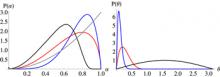# Rochester Institute of TechnologyModeling the Black hole Merger of QSO 3C 186
By Carlos O.Lousto, Yosef Zlochower, Manuela Campanelli
Published in The Astrophysical Journal Letters 841, L28(Wednesday, June 7, 2017)

## Abstract

Recent detailed observations of the radio-loud quasar 3C 186 indicate the possibility that a supermassive recoiling black hole is moving away from the host galaxy at a speed of nearly 2100km/s. If this is the case, we can model the mass ratio and spins of the progenitor binary black hole using the results of numerical relativity simulations. We find that the black holes in the progenitor must have comparable masses with a mass ratio $q=m_1/m_2>1/4$ and the spin of the primary black hole must be $\alpha_2=S_2/m_2^2>0.4$. The final remnant of the merger is bounded by $\alpha_f>0.45$ and at least $4\%$ of the total mass of the binary system is radiated into gravitational waves.  We consider four different pre-merger scenarios that further narrow those values.  Assuming, for instance, a cold accretion driven merger model, we find that the binary had comparable masses with $q=0.70^{+0.29}_{-0.21}$ and the normalized spins of the larger and smaller black holes were $\alpha_2=0.94^{+0.06}_{-0.22}$ and $\alpha_1=0.95^{+0.05}_{-0.09}$.  We can also estimate the final recoiling black hole spin $\alpha_f=0.93^{+0.02}_{-0.03}$ and that the system radiated $9.6^{+0.8}_{-1.4}\%$ of its total mass, making the merger of those black holes the most energetic event ever observed.

## CCRG Authors

Campanelli, ManuelaLousto, Carlos O.Zlochower, Yosef

## Research Areas

Analytic Relativity & Modeling